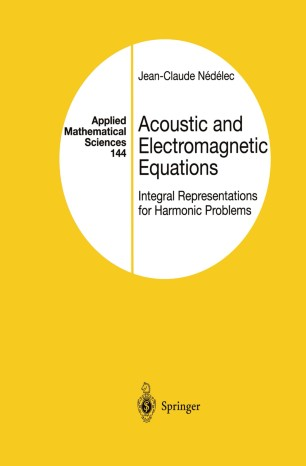# Acoustic and Electromagnetic Equations

## Integral Representations for Harmonic Problems

• Jean-Claude NédélecTextbook

Part of the Applied Mathematical Sciences book series (AMS, volume 144)

1. Front Matter
Pages i-ix
2. Jean-Claude Nédélec
Pages 1-8
3. Jean-Claude Nédélec
Pages 9-109
4. Jean-Claude Nédélec
Pages 110-149
5. Jean-Claude Nédélec
Pages 150-176
6. Jean-Claude Nédélec
Pages 177-300
7. Back Matter
Pages 301-318

### Introduction

This book is devoted to the study of the acoustic wave equation and of the Maxwell system, the two most common wave equations encountered in physics or in engineering. The main goal is to present a detailed analysis of their mathematical and physical properties. Wave equations are time dependent. However, use of the Fourier trans­ form reduces their study to that of harmonic systems: the harmonic Helmholtz equation, in the case of the acoustic equation, or the har­ monic Maxwell system. This book concentrates on the study of these harmonic problems, which are a first step toward the study of more general time-dependent problems. In each case, we give a mathematical setting that allows us to prove existence and uniqueness theorems. We have systematically chosen the use of variational formulations related to considerations of physical energy. We study the integral representations of the solutions. These representa­ tions yield several integral equations. We analyze their essential properties. We introduce variational formulations for these integral equations, which are the basis of most numerical approximations. Different parts of this book were taught for at least ten years by the author at the post-graduate level at Ecole Poly technique and the University of Paris 6, to students in applied mathematics. The actual presentation has been tested on them. I wish to thank them for their active and constructive participation, which has been extremely useful, and I apologize for forcing them to learn some geometry of surfaces.

### Keywords

Helmholtz equation Maxwell equation Wave acoustic wave equation electromagnetic wave mathematical modeling modeling operator wave equation

#### Authors and affiliations

• Jean-Claude Nédélec
• 1
1. 1.Centre de Mathématiques AppliquéesEcole PolytechniquePalaiseau CedexFrance

### Bibliographic information

• DOI https://doi.org/10.1007/978-1-4757-4393-7
• Copyright Information Springer-Verlag New York 2001
• Publisher Name Springer, New York, NY
• eBook Packages
• Print ISBN 978-1-4419-2889-4
• Online ISBN 978-1-4757-4393-7
• Series Print ISSN 0066-5452
• Buy this book on publisher's site
Industry Sectors